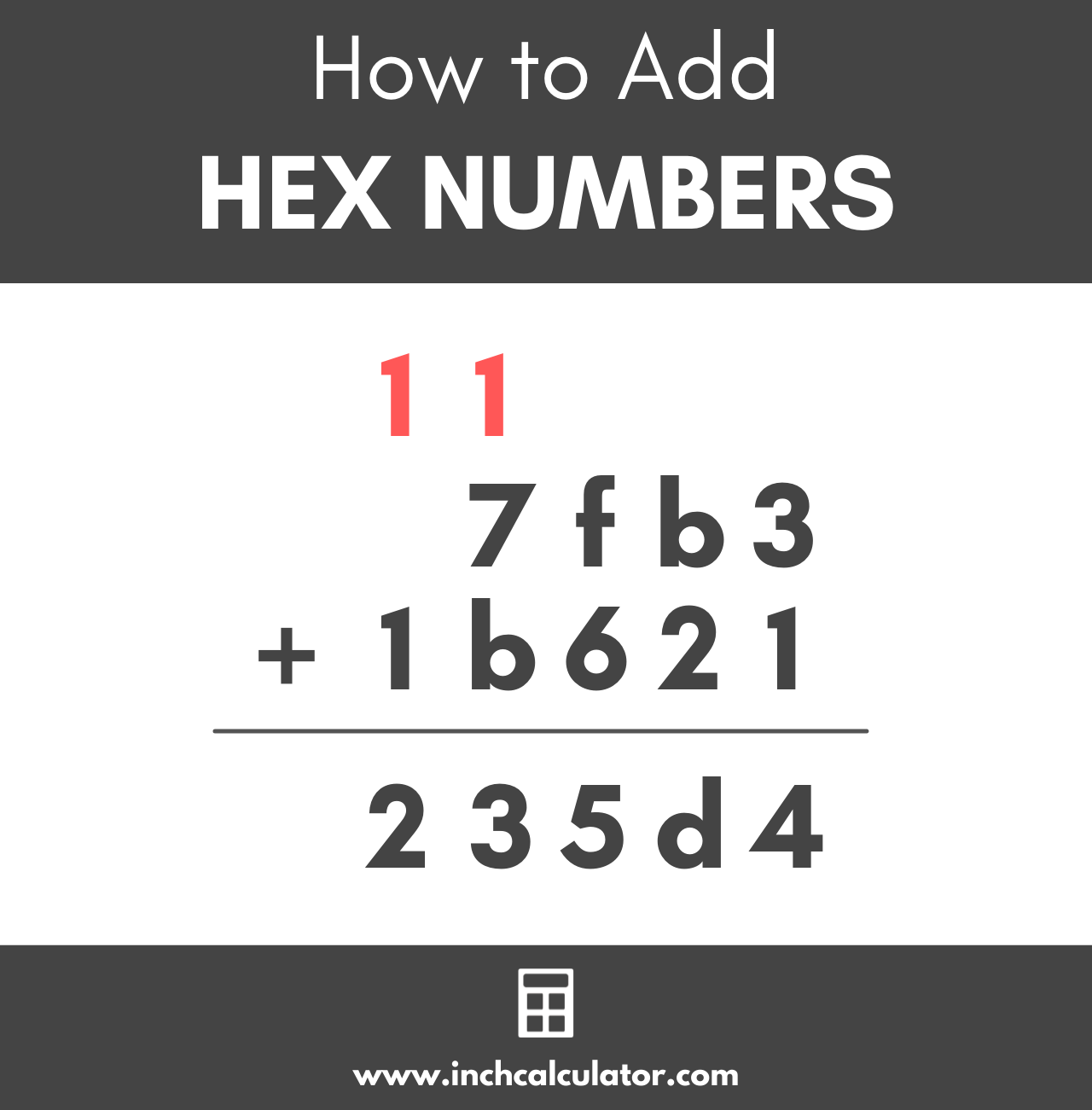Add, subtract, multiply, divide, or convert hexadecimal numbers using the hex calculator below.

### Binary Result:

Learn how we calculated this below

Unlike the decimal system, which is a base 10 number system, the hexadecimal (or hex) system is a base 16 number system and uses the digits 0 through 9 and a through f.To add two hex numbers, write the second number below the first number, aligning the right digits like this:

 7 f b 3 + 1 b 6 2 1

Starting at the right-most number, add the top and bottom values together. In this case, 3 + 1 is equal to 4.

 7 f b 3 + 1 b 6 2 1 4

Then, continue with the second number from the right; b + 2 is equal to d. The digit b in hex is equal to the decimal value of 11, and d is equal to 13. You can also increment the letter by 2 letters.

 7 f b 3 + 1 b 6 2 1 d 4

Moving on to the third number from the right, f + 6 is equal to 15. In our problem, regroup the leading 1 to the top of the problem over the preceding column of digits and move the trailing 5 to the bottom.

 1 7 f b 3 + 1 b 6 2 1 5 d 4

In the next step, add the 1 at the top of the next column with the other values; 1 + 7 + b is equal to 13 in hex. You’ll need to carry the 1 to the top again and the 3 to the bottom like this:

 1 1 7 f b 3 + 1 b 6 2 1 3 5 d 4

The final step is to add the 1 that you just placed at the top with the 1 on the bottom number, which is equal to 2. Therefore, the answer is 235d4.

 1 1 7 f b 3 + 1 b 6 2 1 2 3 5 d 4

## How to Convert a Hex Number

To convert a hexadecimal number to decimal, use the following formula:

decimal number10 = (d0 × 160) + (d1 × 161) + … + (dn – 1 × 16n – 1)

In the formula above, d0 is the hex digit furthest to the right, d1 is the digit one position from the right, and dn – 1 is the digit furthest to the left.